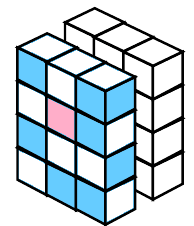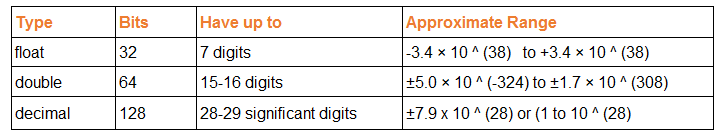Tutorial

# Decimal, Float and Double

In .NET, Decimal, Float, and Double are numeric data types used to represent decimal numbers with varying degrees of precision and range. Each of these data types has its unique characteristics, making them suitable for different scenarios based on precision requirements and memory constraints.

## Decimal

The Decimal data type in .NET is a high-precision floating-point type, also known as a "decimal floating-point." It is commonly used for financial and monetary calculations, where exact precision is crucial to avoid rounding errors. Decimal provides 28-29 significant digits of precision and a range from approximately ±1.0 x 10^-28 to ±7.9 x 10^28.
decimal num1 = 123.456M; decimal num2 = 789.123M; decimal sum = num1 + num2; // Output: 912.579

## FloatThe Float data type is a single-precision floating-point type, often used when a balance between precision and memory usage is necessary. Float provides 7 significant digits of precision and a range from approximately ±1.5 x 10^-45 to ±3.4 x 10^38. However, it may introduce rounding errors when dealing with very small or very large numbers due to its limited precision.
float num1 = 123.456F; float num2 = 789.123F; float sum = num1 + num2; // Output: 912.579

## DoubleThe Double data type is a double-precision floating-point type, offering more significant digits and a larger range compared to Float. It provides 15-16 significant digits of precision and a range from approximately ±5.0 x 10^-324 to ±1.7 x 10^308. Double is often used for general-purpose numerical calculations that require a higher level of precision than Float.
double num1 = 123.456; double num2 = 789.123; double sum = num1 + num2; // Output: 912.579

### Approximate Range## Accuracy

1. Float is less accurate than Double and Decimal.
2. Double is more accurate than Float but less accurate than Decimal.
3. Decimal is more accurate than Float and Double.

### Conclusion

The main differences between Decimal, Float, and Double in .NET are related to precision and range. Decimal offers the highest precision and is best suited for financial and monetary calculations. Float provides a balance between precision and memory usage, while Double offers higher precision than Float and is commonly used for general-purpose numerical calculations. When choosing between these data types, it's essential to consider the specific requirements of your application and the level of precision needed for accurate results.